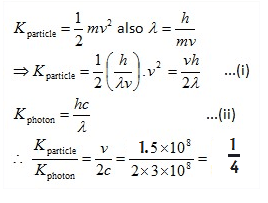# The de-Broglie wavelength of an electron moving with a velocity of 1.5×10^8 m/s is equal to that of a photon. The ratio of kinetic energy of the electron to that of the photon:

Q. The de-Broglie wavelength of an electron moving with a velocity of  is equal to that of a photon.The ratio of kinetic energy of the electron to that of the photon
:

Solution

Matter waves are a central part of the theory of quantum mechanics, being an example of wave–particle duality. All matter can exhibit wave-like behavior. For example, a beam of electrons can be diffracted just like a beam of light or a water wave.

The de Broglie equation is an equation used to describe the wave properties of matter, specifically, the wave nature of the electron: λ = h/mv, where λ is wavelength, h is Planck's constant, m is the mass of a particle, moving at a velocity v. de Broglie suggested that particles can exhibit properties of waves.Thanks for reading The de-Broglie wavelength of an electron moving with a velocity of 1.5×10^8 m/s is equal to that of a photon. The ratio of kinetic energy of the electron to that of the photon:

←Previous
« Prev Post
Next→
Next Post »